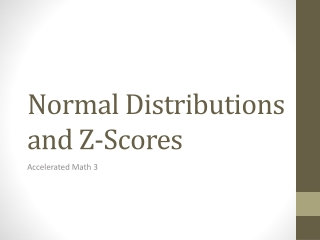DownloadDownload PresentationNormal Distributions and Z-Scores

# Normal Distributions and Z-Scores

Télécharger la présentation## Normal Distributions and Z-Scores

- - - - - - - - - - - - - - - - - - - - - - - - - - - E N D - - - - - - - - - - - - - - - - - - - - - - - - - - -
##### Presentation Transcript

1. Normal Distributions and Z-Scores Accelerated Math 3

2. Normal Distributions Last year we learned about normal distributions and the Empirical Rule.

3. Normal Distributions • The Empirical Rule tells us the probability that a certain range of data falls within one, two, or three standard deviations of the mean. • is the mean and is the standard deviation of the data set.

4. Ex. 1: Word Problem • The data for the SAT is normally distributed with a mean of 1000 and standard deviation 180. What is the probability that a randomly selected student scored between a 640 and 1180?

5. Normal Distributions and Z-Scores • What happens when a value doesn’t quite fit in exactly one, two or three standard deviations? • We can use z-scores and z-tables! • Z-scores tell us exactly how many standard deviations away a value is from the mean and the z-table gives us the probability a value is below that amount.

6. Z-Scores • We can find Z-Scores by using the formula:

7. Example 2: • Looking back at our SAT example, lets find the probability that a student scores under 1200.

8. Example 3: • What if I wanted to know the percent of test takers who score above a 1200?

9. Example 4: • What if I wanted to know the z-score for a score between 900 and 1300?

10. Ex 5: Working Backwards • You run a Tootsie Pop factory and want to make a guarantee that your Tootsie Pops will last a certain number of licks. If your mean is 964 licks with a standard deviation of 51 licks, how many licks should you guarantee your Tootsie Pops will last so that 90% last that long?